Courses

# The Integer Quantum Hall Effect (Part-1) Physics Notes | EduRev

## Physics : The Integer Quantum Hall Effect (Part-1) Physics Notes | EduRev

The document The Integer Quantum Hall Effect (Part-1) Physics Notes | EduRev is a part of the Physics Course Basic Physics for IIT JAM.
All you need of Physics at this link: Physics

The Integer Quantum Hall Effect
In this section we discuss the integer quantum Hall effect. This phenomenon can be understood without taking into account the interactions between electrons. This means that we will assume that the quantum states for a single particle in a magnetic field that we described in Section 1.4 will remain the quantum states when there are many particles present. The only way that one particle knows about the presence of others is through the Pauli exclusion principle: they take up space. In contrast, when we come to discuss the fractional quantum Hall effect in Section 3, the interactions between electrons will play a key role.

Conductivity in Filled Landau Levels
Let’s look at what we know. The experimental data for the Hall resistivity shows a number of plateaux labelled by an integer v. Meanwhile, the energy spectrum forms Landau levels, also labelled by an integer. Each Landau level can accommodate a large, but finite number of electrons.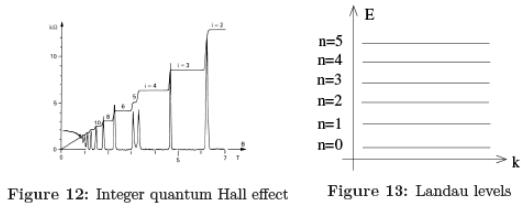It’s tempting to think that these integers are the same: pxy = 2πh/e2v and when precisely v Landau levels are filled. And this is correct.
Let’s first check that this simple guess works. If know that on a plateau, the Hall resistivity takes the value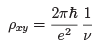with v ∈ Z. But, from our classical calculation in the Drude model, we have the expectation that the Hall conductivity should depend on the density of electrons, n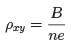Comparing these two expressions, we see that the density needed to get the resistivity of the vth plateau is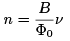(2.1)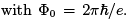This is indeed the density of electrons required to fill v Landau levels.
Further, when v Landau levels are filled, there is a gap in the energy spectrum: to occupy the next state costs an energy hwB where wB = eB/m is the cyclotron frequency. As long as we’re at temperature kBT ≪ hwB, these states will remain empty. When we turn on a small electric field, there’s nowhere for the electrons to move: they’re stuck in place like in an insulator. This means that the scattering time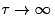and we have pxx = 0 as expected.

Conductivity in Quantum Mechanics: a Baby Version
The above calculation involved a curious mixture of quantum mechanics and the classical Drude mode. We can do better. Here we’ll describe how to compute the conductivity for a single free particle. We’ll derive a more general formula that holds for any many-body quantum system.
We know that the velocity of the particle is given by
mx = p + eA
where pi is the canonical momentum. The current is I = — ex, which means that, in the quantum mechanical picture, the total current is given by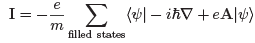It’s best to do these kind of calculations in Landau gauge, A = xBy. We introduce an electric field E in the x-direction so the Hamiltonian is given by (1.23) and the states by (1.24). With the v Landau levels filled, the current in the x-direction is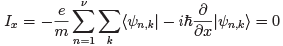This vanishes because it’s computing the momentum expectation value of harmonic oscillator eigenstates. Meanwhile, the current in the y-direction is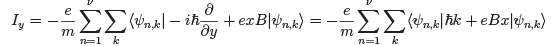The second term above is computing the position expectation value (x) of the eigenstates. But we know from (1.20) and (1.24) that these harmonic oscillator states are shifted from the origin, so that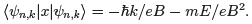The first of these terms cancels the explicit hk term in the expression for Iy. We’re left with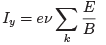(2.2)
The sum over k just gives the number of electrons which we computed in (1.21) to be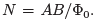We divide through by the area to get the current density J instead of the current I. The upshot of this is that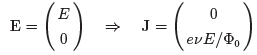Comparing to the de finition of the conductivity tensor (1.6), we have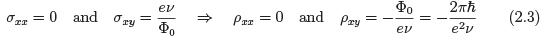This is exactly the conductivity seen on the quantum Hall plateaux. Although the way we’ve set up our computation we get a negative Hall resistivity rather than positive; for a magnetic field in the opposite direction, you get the other sign.

Edge Modes
There are a couple of aspects of the story which the simple description above does not capture. One of these is the role played by disorder; we describe this in Section 2.2.1. The other is the special importance of modes at the edge of the system. Here we describe some basic facts about edge modes; we’ll devote Section 6 to a more detailed discussion of edge modes in the fractional quantum Hall systems.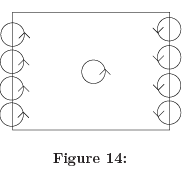The fact that something special happens along the edge of a quantum Hall system can be seen even classically. Consider particles moving in circles in a magnetic field. For a fixed magnetic field, all particle motion is in one direction, say anti-clockwise. Near the edge of the sample, the orbits must collide with the boundary. As all motion is anti-clockwise, the only option open to these particles is to bounce back. The result is a skipping motion in which the particles along the one-dimensional boundary move only in a single direction, as shown in the figure. A particle restricted to move in a single direction along a line is said to be chiral. Particles move in one direction on one side of the sample, and in the other direction on the other side of the sample. We say that the particles have opposite chirality on the two sides. This ensures that the net current, in the absence of an electric field, vanishes.
We can also see how the edge modes appear in the quantum theory. The edge of the sample is modelled by a potential which rises steeply as shown in the figure. We'll work in Landau gauge and consider a rectangular geometry which is finite only in the x-direction, which we model by V(x). The Hamiltonian is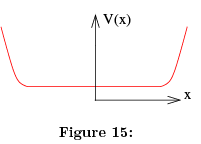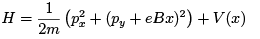In the absence of the potential, we know that the wavefunctions are Gaussian of width lB. If the potential is smooth over distance scales lB, then, for each state, we can Taylor expand the potential around its location X. Each wavefunction then experiences the potential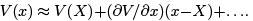We drop quadratic terms and, of course, the constant term can be neglected. We're left with a linear potential which is exactly what we solved in Section 1.4.2 when we discussed Landau levels in a background electric field. The result is a drift velocity in the y-direction (1.26), now given by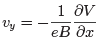Each wavefunction, labelled by momentum k, sits at a different x position,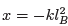and has a different drift velocity. In particular, the modes at each edge are both chiral, travelling in opposite directions: vy > 0 on the left, and vy < 0 on the right. This agrees with the classical result of skipping orbits.
Having a chiral mode is rather special. In fact, there's a theorem which says that you can’t have charged chiral particles moving along a wire; there has to be particles which can move in the opposite direction as well. In the language of field theory, this follows from what’s called the chiral anomaly. In the language of condensed matter physics, with particles moving on a lattice, it follows from the Nielsen-Ninomiya theorem. The reason that the simple example of a particle in a magnetic field avoids these theorems is because the chiral fermions live on the boundary of a two-dimensional system, rather than in a one-dimensional wire. This is part of a general story: there are physical phenomena which can only take place on the boundary of a system. This story plays a prominent role in the study of materials called topological insulators.
Let’s now look at what happens when we fill the available states. We do this by introducing a chemical potential. The states are labelled by y-momentum hk but, as we’ve seen, this can equally well be thought of as the position of the state in the x-direction. This means that we’re justified in drawing the filled states like this: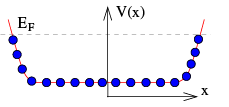From our usual understanding of insulators and conductors, we would say that the bulk of the material is an insulator (because all the states in the band are filled) but the edge of the material is a metal. We can also think about currents in this language. We simply introduce a potential difference Δμ on the two sides of the sample. This means that we fill up more states on the right-hand edge than on the left-hand edge, like this: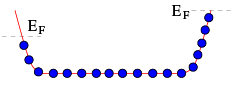To compute the resulting current we simply need to sum over all filled states. But, at the level of our approximation, this is the same as integrating over x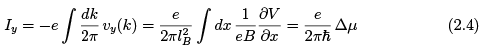The Hall voltage is eVH = Δμ, giving us the Hall conductivity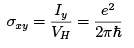(2.5)
which is indeed the expected conductivity for a single Landau level.
The picture above suggests that the current is carried entirely by the edge states, since the bulk Landau level is flat so these states carry no current. Indeed, you can sometimes read this argument in the literature. But it's a little too fast: in fact, it's even in conflict with the computation that we did previously, where (2.2) shows that all states contribute equally to the current. That’s because this calculation included the fact that the Landau levels are tilted by an electric field, so that the effective potential and the filled states looked more like this: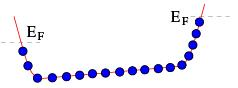Now the current is shared among all of the states. However, the nice thing about the calculation (2.4) is that it doesn’t matter what shape the potential V takes. As long as it is smooth enough, the resulting Hall conductivity remains quantised as (2.5). For example, you could consider the random potential like this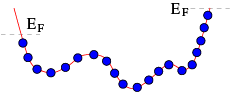and you still get the quantised answer (2.4) as long as the random potential V(x) doesn’t extend above EF. As we will describe in Section 2.2.1, these kinds of random potentials introduce another ingredient that is crucial in understanding the quantised Hall plateaux.
Everything we’ve described above holds for a single Landau level. It’s easily generalised to multiple Landau levels. As long as the chemical potential EF lies between Landau levels, we have n filled Landau levels, like this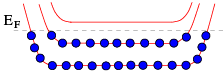Correspondingly, there are n types of chiral mode on each edge.
A second reason why chiral modes are special is that it’s hard to disrupt them. If you add impurities to any system, they will scatter electrons. Typically such scattering makes the electrons bounce around in random directions and the net effect is often that the electrons don’t get very far at all. But for chiral modes this isn’t possible simply because all states move in the same direction. If you want to scatter a left-moving electron into a right-moving electron then it has to cross the entire sample. That’s a long way for an electron and, correspondingly, such scattering is highly suppressed. It means that currents carried by chiral modes are immune to impurities. However, as we will now see, the impurities do play an important role in the emergence of the Hall plateaux.

Offer running on EduRev: Apply code STAYHOME200 to get INR 200 off on our premium plan EduRev Infinity!

## Basic Physics for IIT JAM

109 videos|157 docs|94 tests

,

,

,

,

,

,

,

,

,

,

,

,

,

,

,

,

,

,

,

,

,

;